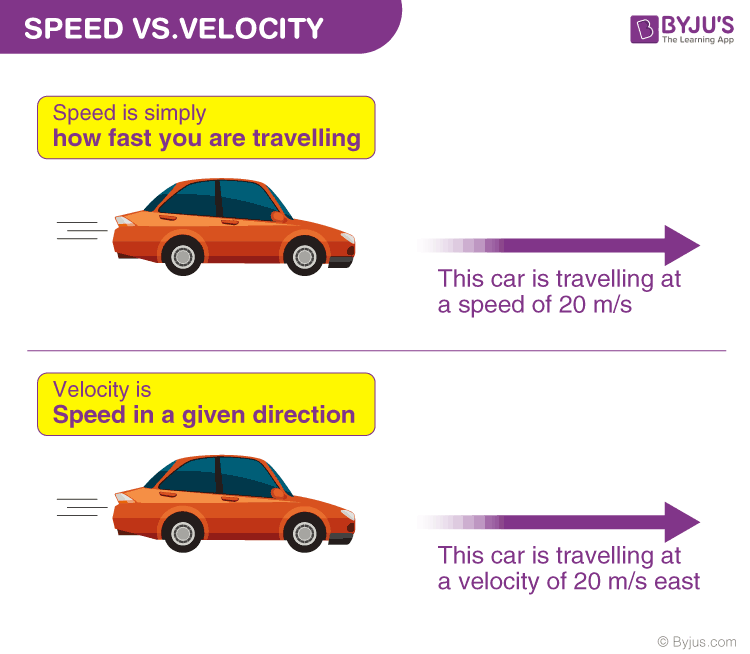# Average Velocity

When an object moves, its position changes with time. But have you wondered how fast an object changes its position with time or in what direction? To describe this, we use average velocity. In this article, let us familiarize ourselves with average velocity

## What is Average Velocity?

Average velocity is a vector quantity. Average velocity is defined as the change in position or displacement (∆x) divided by the time intervals (∆t) in which the displacement occurs.The average velocity can be positive or negative depending upon the sign of the displacement. The SI unit of average velocity is meters per second (m/s or ms-1).

### Finding Average Velocity

To calculate the average velocity, we need to divide the total displacement by the total time elapsed as follows:

$$\begin{array}{l}\bar{v}=\frac{\Delta x}{\Delta t}=\frac{x_{f}-x_{0}}{t_{f}-t_{0}}\end{array}$$

Where ΔV is the average velocity, Δx is the displacement, Δt is the total time, xf and x0 are the initial and final positions, tf and t0 are the starting and ending times.

If the starting time is zero, then the formula becomes:

$$\begin{array}{l}\bar{v}=\frac{\Delta x}{t}\end{array}$$

Average velocity in a distance-time graph is shown below:### Average Velocity Example

During a 4 s time interval, the runner’s position changes from x1 = 60 m to x2= 40 m. What is the runner’s average velocity?

Solution:

The change in the displacement of the runner is calculated as follows:

Δx = x2 – x1 = 40 m – 60 m = -20 cm

Δt = 4 s

vaverage = Δx/Δt = -20 m/ 4 s = -5 m/s

## Difference Between Average Speed and Average Velocity

Average velocity involves only the displacement of the object. However, the magnitude of the displacement may be different from the actual path length. For this reason, to describe the rate of motion over a path, we use average speed.

Average speed is defined as the total path length travelled divided by the total time interval of the motion. Unlike average velocity, the average speed is a scalar quantity. Average speed does not tell us the direction of motion; thus, it is always positive.

For better understanding, let us look at the example below:The average speed of the above car is 20 m/s, but the average velocity is 20 m/s, east.

### When is the average velocity zero?

For instance, if a remote-controlled car is racing from Point A to Point B and back to Point A, there is no overall car displacement. In that case, the speed of the car can be measured but not its velocity. If the same car moves from Point A to Point B and stays there, there is definite displacement in a certain direction. It can be measured in such cases. In simpler words, average velocity is just the average speed with a direction. If a trip starts and ends at the same point, the total displacement is zero, so the average velocity is zero.

Here is a video that provides a detailed explanation of average speed and average velocity with animations!Related Articles

## Frequently Asked Questions – FAQs

### What is Average Velocity?

Average velocity is defined as the change in position or displacement divided by the time intervals in which the displacement occurs.

### How can average velocity be zero?

If a trip starts and ends at the same point, the total displacement is zero, so the average velocity is zero.

### Can average velocity be zero?

The average velocity can be positive or negative depending upon the sign of the displacement.

### Is Average velocity a vector or a scalar?

Average velocity is a vector quantity.

### How can average velocity be identified?

To calculate the average velocity, we need to divide the total displacement by the total time elapsed.

Stay tuned to BYJU’S and Fall in Love with Learning!

Test your knowledge on Average Velocity

1. Amanuel

It is nice encouraged me to see in other lesson.

2. I have learned a lot! This has helped me understand graphing a little bit more. 🙂

3. Mridul Sharma

I have learnt a lot. Its very easy to understand its languageand every time it clears all my doubts

4. Anvitha

Good content